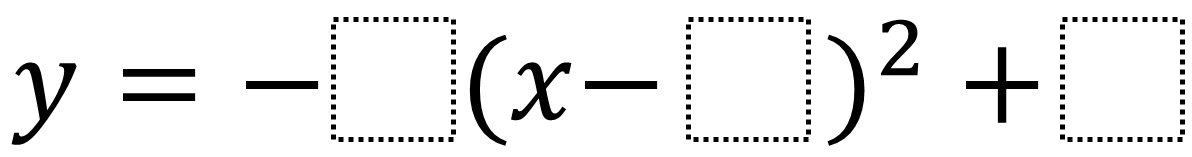Home > High School: Functions > Maximum Value of a Quadratic in Vertex Form

# Maximum Value of a Quadratic in Vertex Form

Directions: Create a quadratic equation with the greatest possible maximum value using the digits 1 through 9, no more than one time each.### Hint

Where is the best place to put the 9? How does each box affect the graph’s behavior?

There are many solutions but a 9 must go into the last term as the k-value. That affects its y-axis position while the the a and h terms affect its width and x-axis position.  Play with the sliders on this Desmos graph for a hands on way of understanding how the a, x, and k terms affect the quadratic: https://www.desmos.com/calculator/uffgbapfzf

Source: Robert Kaplinsky

## Creating Sequences

Directions: Using the digits 1-9, at most one time each, complete the first three terms …

1.•